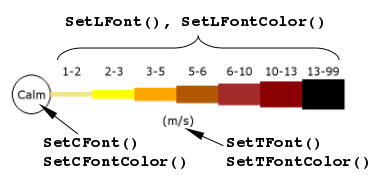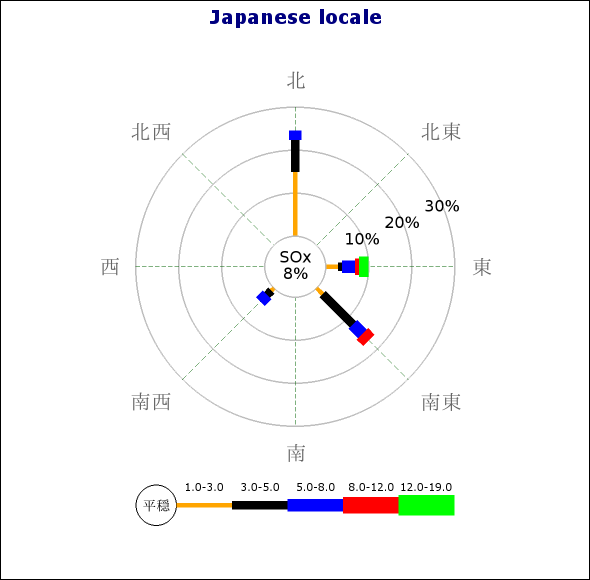## 21.3. Formatting the plot

### 21.3.1. Positioning the plot

The position of the graph can be controlled with the method

• `WindrosePlot::SetPos(\$aXPos,\$aYPos)`

The position can be specified as either absolute position (in pixels) or as a fraction of width and height. The anchor for the position is the center of the windrose plot. For example, the following line centers the plot in the graph

 ```1 ``` `\$windplot->SetPos(0.5,0.5);`

### 21.3.2. Specifying the size of the plot

In the same way as for the position the size of the windrose plot can be specified as either an absolute value or as a fraction of the minimum of width and height. The size specified refers to the diameter of the plot.

The size is controlled with the the method

• `WindrosePlot::SetSize(\$aDiameter)`

as in

 ```1 ``` `\$windplot->SetSize(200);`

The code above sets the diameter of the plot to 200 pixels, setting the value to 0.7 would set the diameter to 0.7 * min(WIDTH,HEIGHT) where width and height are the size of the graph.

### 21.3.3. Specifying the size of the Zero-circle (middle circle)

The size of the middle circle (the zero-range circle) can be adjusted with the method

• `WindrosePlot::SetZCircleSize(\$aDiameter)`

`\$aDiameter`, The diameter can be specified as either an absolute integer value or as a fraction of the windrose plot diameter.

For example the following line sets the size to 1/3 of the windrose plot.

 ```1 ``` `\$windplot->SetZCircleSize(1/3);`

### 21.3.4. Formatting the plot legend

The legend is displayed in a fixed position just below the windrose plot as can be seen in all the previous examples.

All legend methods is accessed through the legend property of the windrose plot as in

 ```1 ``` `\$windroseplot->legend->SetFont(...);`

There are four basic attributes that can be customizable for the legend as described in the following paragraphs.

#### Specifying legend fonts

To allow for maximum flexibility font can be specified for both the numerical ranges as well as for the text under the legend. In order to avoid making the legend to wide it is often desirable to use a smaller font for the ranges and a slightly larger font for the actual text under the legend (as well as inside the "calm" circle - the indicator for bucket 0).

The fonts for the legend is adjusted by the following methods

• `SetTFont()`, The text below the legend

• `SetLFont()`, The font for the labels

• `SetCFont()`, The font for the label inside the "calm" circle

• `SetFont()`, Sets all the font areas above the same font. Note that if this method is called after one of the individual settings previous specified (e.g. `SetCFont()`) they will be overwritten.

The colors for the fonts are specified in an analogue way by using the methods

• `SetTFontColor()`

• `SetLFontColor()`

• `SetCFontColor()`

• `SetFontColor()`

Figure 21.10 explains visually how these methods are applied

Figure 21.10. Windrose legend methodsFigure 21.11 illustrates how two fonts were used for the legend. For the circle we have use a Chinese font (The Kanji characters roughly means "Chinese language")

### Caution

The source file (as every source file in the library) is encoded in utf-8. For technical reasons some browsers might not render the Chinese characters correctly when the source file is viewed.

Figure 21.11. Using chinese fonts `(windrose_ex6.1.php)`#### Setting text and colors for the Zero-circle

The "Calm" circle can have it's size, line weight and color adjusted. In addition (as was shown in Figure 21.11) specify any text within the circle. By default the text "Calm" is printed inside the circle. The following methods are used to adjust these properties.

• `SetCircleWeight(\$aWeight)`

• `SetCircleRadius(\$aRadius)`

• `SetCircleColor(\$aColor)`

• `SetCircleText(\$aTxt)`

#### Specifying number formats for ranges

For the ranges it is possible to adjust how the numbers are formatted with a general format string (in the style of a `printf()` format string). This would allow, for example, to choose the number of decimal values to show in the legend. The format string is specified with a call to

• `Legend::SetFormat(\$aFmt)`.

The format will be applied to both the lower and upper range value. This means that the actual legend range text in the legend will be printed as [number format]-[number format].

The following example shows how to make sure the ranges are displayed as integers

 ```1 ``` `\$wp->legend->SetFormat('%d');`

#### Specifying legend bottom text

Finally it is also possible to specify a text at the bottom of the legend with a call to

• `Legend::SetText(\$aTxt)`. The text will be displayed just underneath the legend as for example, shown in Figure 21.11

#### Specifying the numeric values displayed for the ranges

The values displayed in the legend for the ranges can be adjusted with a call to

• `WindRosePlot::SetRanges(\$aRanges)`. By default the ranges are set to 0,1,2,3,5,6,10,13.5,99.0, this means that the ranges printed will be 1-2, 2-3, 3-5, ....

The following example shows how to change this to the ranges 0.000-0.001, 0.001-0.003, 0.003-0.005

 ```1 2 ``` ```\$windplot->SetRanges(array(0.001,0.003,0.005)); \$windplot->SetFormat('%.3f');```

As can be seen the first range (bucket 0) is not really directly printed. It is by default indicated by the circle to the left in the legend.

In the example lines above we also changed the format to display the decimals. Without this the default is only to show one decimal and that would show all ranges as 0.0. An example of how this might look can be seen in figure 10 below.

### 21.3.5. Adjusting the grid colors

The colors of both the radial grid and the circular grid can be adjusted. This is done with the method

• `WindrosePlot::SetGridColor(\$aColor1,\$aColor2)`

The first argument specifies the grid circle color and the second argument specifies the radial grid colors.

### 21.3.6. Adjusting the weight of the grid circles

To adjust the weight (thickness) of the grid lines (both circle and radial lines) the following method is used

• `WindrosePlot::SetGridWeight(\$aWeight1,\$aWeight2)`

The first argument specifies the weight of the circle and the second the weight of the radial lines.

### Note

It is possible to have individual colors of the radial grid lines (and line style &weight) by using any of the methods

• `SetRadialColors()`

• `SetRadialStyles()`

• `SetRadialWeight()`

### Note

Due to technical and performance limitations circle weight can only be specified in the range [1,3] (inclusively).

### 21.3.7. Adding a box around the plot

Finally we remember that it is possible to add a rectangular box around the plot by using the method

• ```WindrosePlot::SetBox(\$aColor='black', \$aWeight=1, \$aStyle='solid', \$aShow=true)```

an example of this can be seen in Figure 21.7.

### 21.3.8. Adjusting the size of the center zero circle

The size of the center circle can be manually adjusted as either specified in absolute number of pixels or as fractions of the windrose plot (not the entire windrose graph). This is done with the method

• `WindrosePlot::SetZCircleSize(\$aSize)`

### 21.3.9. Localizing the default names for the compass directions

By default the compass cardinal directions are presented in English locale using standard denominations for the 16 compass directions.

Localizing these names are done with a call to the method

• `WindrosePlot::SetCompassLabels(\$aLabels)`

The order of the names for the directions should start with the corresponding name for "East" and then continue counter-clockwise all the way to "East North East". Adjusting the names for the compass directions means two things:

• If there is named direction in the data (as key) that key must now also be in localized form.

• The displayed names for the compass direction will be shown in localized form.

For example, the code snippet below shows how to localize the compass directions into Swedish.

 ```1 2 3 4 5 6 7 8 9 10 ``` ```... \$data = array( 'sso' => array(12,8,2,3), 6 => array(5,4,4,5,4), ); \$se_CompassLbl = array('O','ONO','NO','NNO','N','NNV','NV','VNV','V','VSV','SV','SSV','S','SSO','SO','OSO'); .... \$windroseplot->SetCompassLabels(\$se_CompassLbl); ...```

and the following shows how to make a localization into Japanese

### Caution

The source file (as every source file in the library) is encoded in utf-8. For technical reasons some browsers might not render the Japanese characters correctly when the source file is viewed.

Figure 21.12. Japanese locale `(windrose_ex7.1.php)`### 21.3.10. Adjusting the formatting of the windrose plot legs

#### Specifying range colors

To adjust the colors used for each range in the plot the method

`WindrosePlot::SetRangeColors(\$aRangeColors)`

The following example shows how to set a red-brown color scale

 ```1 2 ``` ```\$rangeColors = array('khaki','yellow','orange','orange:0.7','brown','darkred','black'); \$windplot->SetRangeColors(\$rangeColors);```

#### Specifying the width of the plot "legs"

The weight (width) of the legs used for each range in the plot is by default set to the range 2,4,6,8,10,12,14,16,18,20 pixels.

This can be adjusted with a call to the method

• `WindrosePlot::SetRangeWeights(\$aWeights)`

If fewer weights than ranges used are supplied the ranges will wrap-around. The following example shows how to set a larger difference in size for the legs between the ranges.

 ```1 ``` `\$windplot->SetRangeWeights(array(2,6,10,14,18));`